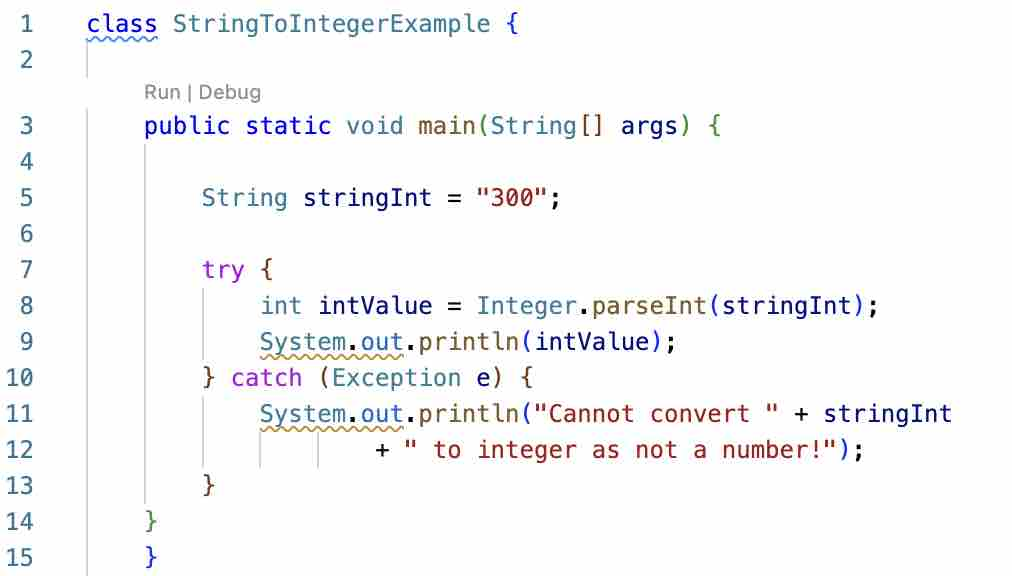# Convert String to int in JavaIn order to convert a String to int in Java Programming language you can follow the below code examples,

1. Using Interger.parseInt(String str) method
``````class StringToIntegerExample {

public static void main(String[] args) {

String stringInt = "25"; //A String variable holding an int value.
int intValue = Integer.parseInt(stringInt);

System.out.println(intValue); //Prints out 25
}
}``````

But its always better to surround this code with a try-catch block as it can throw NumberFormatException if your String object is not holding an integer value, try replacing stringInt from 25 to A25, run the code and you will get the below error stack trace.

``````Exception in thread "main" java.lang.NumberFormatException: For input string: "A25"
at java.base/java.lang.NumberFormatException.forInputString(NumberFormatException.java:65)
at java.base/java.lang.Integer.parseInt(Integer.java:652)
at java.base/java.lang.Integer.parseInt(Integer.java:770)
at StringToIntegerExample.main(Client.java:6)``````

Let's put the above code in try/catch block:

``````class StringToIntegerExample {

public static void main(String[] args) {

String stringInt = "A25";
try {
int intValue = Integer.parseInt(stringInt);
System.out.println(intValue);
} catch (Exception e) {
System.out.println("Cannot convert " + stringInt
+ " to integer as not a number!");
}
}
}``````

2. Using Integer.valueOf(String str) method
``````class StringToIntegerExample {

/**
* Example:
* String to integer using
* Integer.valueOf(String str)
*
* @param args
*/
public static void main(String[] args) {

String stringInt = "300";

try {
int intValue = Integer.valueOf(stringInt);
System.out.println(intValue);
} catch (Exception e) {
System.out.println("Cannot convert " + stringInt
+ " to integer as not a number!");
}
}
}``````
-

Have Questions? Post them here!